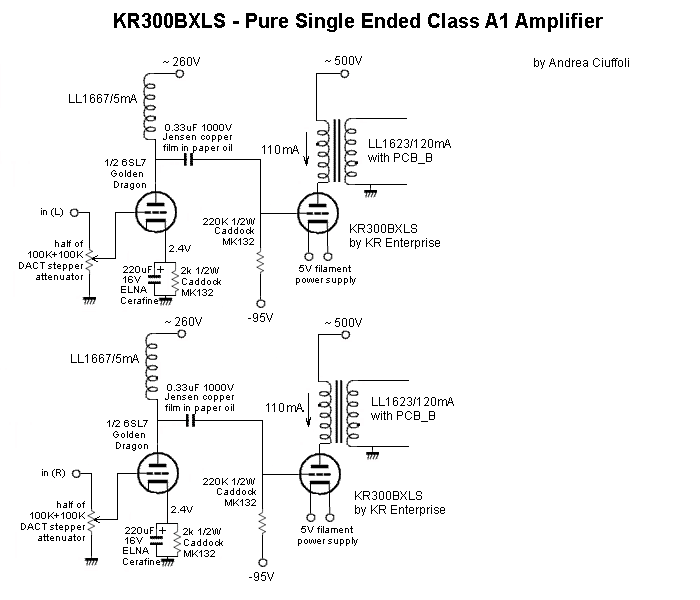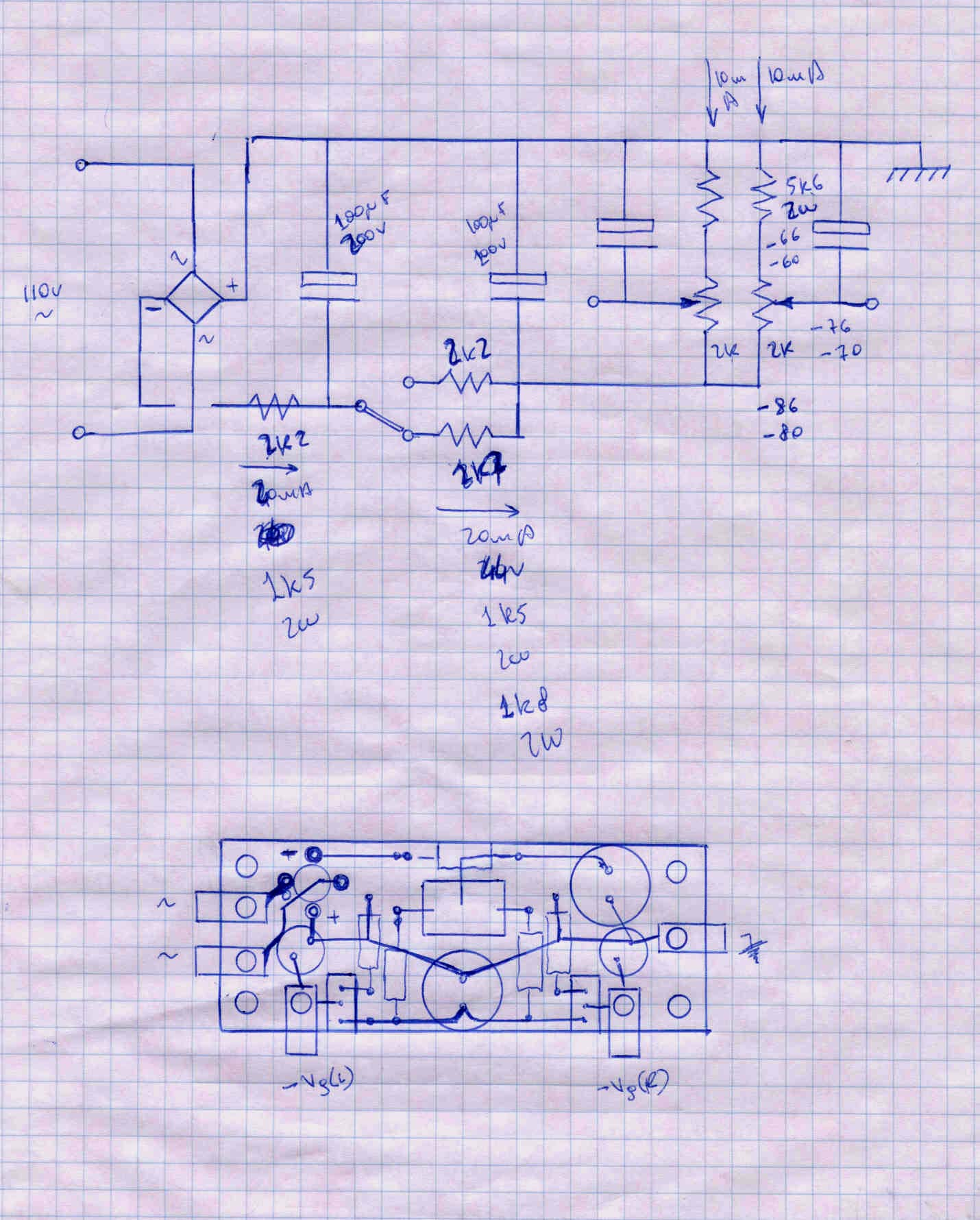back to www.audiodesignguide.com To get more information contact me at: webmaster@audiodesignguide.com

# the 300BXLS Single Ended Amplifier by Andrea Ciuffoli - LAST UPDATE 18 Oct 2001

 ATTENTION: this project is in design phase so any part could change in the near future.

## Introduction

This project is created to integrate in a single component all the experiences made on the previous projects.
Here no compromise has been accepted in any stage.

The main results and targets should match these items:

• top sonic performances
• 2 stages only from DAC chip to the loudspeakers
• very low distortion
• good damping factor with an output resistance near 1.5ohm
• very good low frequency response about 10Hz (-3db)
• no electrolitycs on the power supply but only paper in oil
• no voltage duplicator on the power supply
• soft power supply using indirect heating vacuum diodes (6D22S Svetlana or GZ34)

ComponentsThe KR300BXLS is an ultra-linear, low frequency, high power triode tube capable of producing 15-25 watts of pure class-A power.  The KR300BXLS may be used in 300B amplifiers, however you will still obtain only 8 watts.  Therefore we recommend the KR300B for these applications.   However, if your amplifier has adjustable bias, the KR300BXLS will provide more power.  Increasing the plate voltage and current will result in higher power than is obtainable with other 300Bs.  Consult your equipment manufacturer before increasing the power of your amplifier. The KR300BXLS features a new ribbon filament construction and the KR Electronics exclusive patent design with 32 cathodes. As with all KR Electronics vacuum tubes, the KR300BXLS possesses a vacuum of 10-8 Torr, a maximum grid current of 2.0 microampere and absolutely constant emission over the life of the tube.  All KR Electronics vacuum tubes are warranted for a period of 2 years. Information from:

All the audio transformers used in this project are Lundahl products and the distributor list is available here

• 2 x LL1623/120mA + PCB_B     Lundahl output transformers     (price about 148\$ each)
• 2 x LL1667/5mA                         Lundahl anodic choke
• 2 x LL1651                                  Lundahl anodic chokes
• 2 x LL1638/8H 200mA                Lundahl power supply inductances (price about 65\$ each)
• 3 x MQA-3G3                             Jensen 100uF double metallized paper and polypropylene

•                                                     dielectric impregnated with mineral oil.
• 4 x 534 100ohm                           Spectrol precision wire wound multi-turn potentiometers
• 2 x 64W 2Kohm                          Spectrol multiturn cermet trimmer

•Lundahl is one of the world´s leading manufacturer of audio transformers used in many professional studio recording and radio stations so it has great experience and all these products use a custom audio C-core.

I am using a DACT stepper attenuator instead of the normal ALPS or NOBLE solution, because the the sound is clearer. A good review of these components can be found on the article published in Hi-Fi World.

The input stage is the very good Golden Dragon 6SL7.

The vacuum diodes used are the 6D22S Svetlana to use with the PC509 connector.About passive components types,  I don't leave many choices:  Caddock resistances on cathode, ELNA Cerafine capacitors on cathode.
In all my previous projects I have used in the power supply the ELNA Cerafine capacitors with a very high sonic performances and compact size.
If we skip any economic aspect and if there are no size problems the first component is a paper in oil capacitor.
In comparation test the paper in oil are best of the ELNA Cerafine or other electrolityc type.
It is important to find new products such as Jensen and not old stock.

About wire; forget the teflon ! Use only stranded tinned copper wire with pvc insulator, and for loudspeakers use the same.

A very good quality stranded tinned copper wire could be bought directly from E-Z-HOOK that carries an extensive line of fine stranded and extra flexible wire.

## Design

As in all my amplifier projects my first point is get a very good damping factor to have good bass frequency and good control of loudspeakers.
To get good damping is necessary to use upper impedance of the output transformer but this ofcourse reduces the output power.
In this project for the 300BXLS I have used a 5600ohm on 8ohm that mean a turn ratio of sqrt(5600/8) = 26.45  instead of the suggested  2000ohm that means a turn ratio of sqrt(2000/8) = 15.8 or sqrt(2000/4) = 22.4 depending on load (4 or 8ohm).

The first step in the design is plot the line of the maximun power dissipated by the tube.
I have ploted the line relative to a power 10% less than max power 58.5W.
For each anode voltage has been calculated the relative current at this power with:

P0 = Pd / Va = 58.5W/585 = 100mA
P1 = Pd / Va = 58.5W/520 = 112mA
P2 = Pd / Va = 58.5W/455 = 129mA
P3 = Pd / Va = 58.5W/390 = 150mA
P4 = Pd / Va = 58.5W/325 = 180mA
P5 = Pd / Va = 58.5W/260 = 225mA

I have choicen the operation point PA (487V and 112mA) for this first test.

Pa = Va * Ia = 487V * 112mA = 54.8W about 55W of power dissipated on tube

Now to draw the output line we need to calculate the end point on current axe with:

PB = Va / Rl + Ia = 487V / 5600ohm + 112.5mA = 199mA  on 8ohm
PC = Va / Rl + Ia = 487V / 2800ohm + 112.5mA = 286mA  on 4ohm

click on the image to enlarge.

You can calculate the output power for the two power supply voltage on 4-8ohm load with:

1) (406 - 142) / sqrt(5600 / 8) = 264V / 26.45  = 10Vp = 7.1Vrms => 6.3W on 8ohm in pure class A1 operation

2) (406 - 170) / sqrt(5600 / 8) = 236V / 26.45  = 8.9Vp = 6.3Vrms => 10W on 4ohm in pure class A1 operation

3) (503 - 150) / sqrt(5600 / 8) = 353V / 26.45  = 13.35Vp = 9.46Vrms => 11.2W on 8ohm in pure class A1 operation

4) (503 - 170) / sqrt(5600 / 8) = 333V / 26.45  = 12.6Vp = 9Vrms => 20W on 4ohm in pure class A1 operation

And the bias current for each of previous points with:

Ia(min) = 1.41 * sqrt(Pout/Rload)/(turn ratio) = 1.41 * sqrt(Pout/Rload)/sqrt(5600/8) = 1.41 * sqrt(Pout/Rload)/26.45

1) Ia(min) = 1.14 * sqrt(6.3W/8ohm)/26.45 = 47mA

2) Ia(min) = 1.41 * sqrt(10W/4ohm)/26.45 = 84mA

3) Ia(min) = 1.41 * sqrt(11.2W/8ohm)/26.45 = 64mA

4) Ia(min) = 1.41 * sqrt(20W/4ohm)/26.45 = 120mA

The LL1623/120mA has an inductance of 23H (see datasheet on http://www.lundahl.se/pdfs/datash/1620_3_7.pdf) and we can calculate the low frequency cut-off with:

Ft(-3db) = R / (2 * pi * L) = (Ra + Rt) / (2 * 3.14 * L) = (600 + 164) / (2 * 3.14 * 23) = 5.3Hz

The LL1667/15mA has an inductance of 270H (see datasheet on http://www.lundahl.se/pdfs/datash/1667_68.pdf) so the LL1667/5mA will have an inductance of 270H * (15mA / 5mA) = 270 * 3 = 810H and we can calculate the low frequency cut-off with:

Ft(-3db) = R / (2 * pi * L) = (Ra + Rt) / (2 * 3.14 * L) = (44000 + 2400) / (2 * 3.14 * 810) = 9.12Hz

The low frequency cut-off of the  interstage capacitor is calculated with:

Ft(-3db) = 1 / (2 * pi * C * R) = 1 / (2 * 3.14 * 0.33uF * 220K) = 1 / (2 * 3.14 * 0.33E-6 * 220000) = 2.2Hz

The dissipated power of tubes has been calculated with:

Pd(6sl7) = I * V = (2.4V/2k) * 260V = (2.4/2000) * 260 = 0.312W and the max limit is 1W

Pd(300bxls) = I * V = 110mA * 500V = 0.11 * 500 = 55W and the max limit is 65W

The power supply current and power used for the anodic volatge is calculated with:

I = 2 * (2.4V/2K) + 2 * 110mA = 0,2224A

P = 500V * 0.2224 = 111W

The main schematicPower supply

Before inserting the 300BXLS tubes in the sockets switch on the amplifier without the fuse and set the trimmers to get about -90V from the grids of the 300BXLS and the ground.
After switching off, insert the fuse, insert the 300BXLS and switch on the amp.

Here is the first version of the schematic using the 5709XH mains transformers by Antrim custom made on Studio-M specification.another choice with a custom transformerHere the suggested connection maries and secondaries using the 5709XH mains transformers by Antrim custum made on Studio-M specification.
This connection allow the get both anodic voltage about 520V and bias voltage about 120V.

Digital Input stage

This project gives a first class sonic performance but this can be easily compromised by a  bad input signal so I suggest you don't use preampl. and use one of my DAC projects instead.
Using one of my top DAC projects all the DIYer's will reach the same sonic result.
The DAC normally included into commercial CDPlayer (even these of significant price) have a rather low performance because the common usage of high NFB operational amplifiers "destroy" the sound given compression effects and poor soundstage stability.

Sonic results

Layout views

click on the images to get real scale

Layout size

click on the images to get real scale

Bias board

click on the images to get real scale